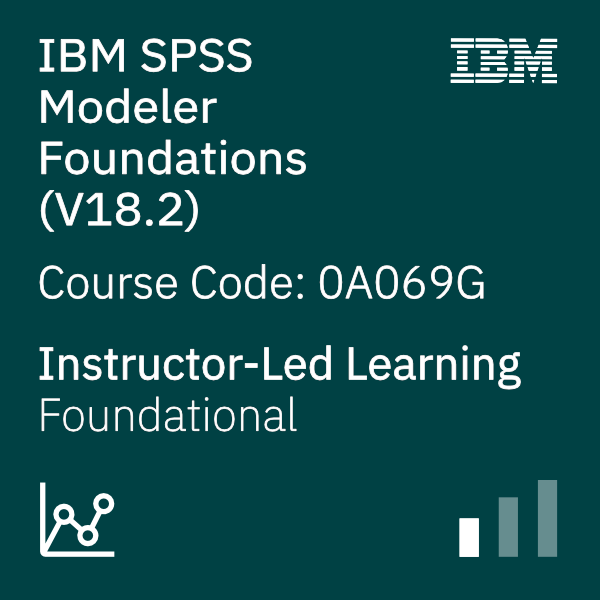# Overview

This course provides the foundations of using IBM SPSS Modeler and introduces the participant to data science. The principles and practice of data science are illustrated using the CRISP-DM methodology. The course provides training in the basics of how to import, explore, and prepare data with IBM SPSS Modeler v18.2, and introduces the student to modeling.

# Audience

• Data scientists
• Clients who are new to IBM SPSS Modeler or want to find out more about using it

# Objective

Introduction to IBM SPSS Modeler
• Introduction to data science
• Describe the CRISP-DM methodology
• Introduction to IBM SPSS Modeler
• Build models and apply them to new data

Collect initial data
• Describe field storage
• Describe field measurement level
• Import from various data formats
• Export to various data formats

Understand the data
• Audit the data
• Check for invalid values
• Take action for invalid values
• Define blanks

Set the unit of analysis
• Remove duplicates
• Aggregate data
• Transform nominal fields into flags
• Restructure data

Integrate data
• Append datasets
• Merge datasets
• Sample records

Transform fields
• Use the Control Language for Expression Manipulation
• Derive fields
• Reclassify fields
• Bin fields

Further field transformations
• Use functions
• Replace field values
• Transform distributions

Examine relationships
• Examine the relationship between two categorical fields
• Examine the relationship between a categorical and continuous field
• Examine the relationship between two continuous fields

Introduction to modeling
• Describe modeling objectives
• Create supervised models
• Create segmentation models

Improve efficiency
• Use database scalability by SQL pushback
• Process outliers and missing values with the Data Audit node
• Use the Set Globals node
• Use parameters
• Use looping and conditional execution

Show details

# Course Outline

Introduction to IBM SPSS Modeler
• Introduction to data science
• Describe the CRISP-DM methodology
• Introduction to IBM SPSS Modeler
• Build models and apply them to new data

Collect initial data
• Describe field storage
• Describe field measurement level
• Import from various data formats
• Export to various data formats

Understand the data
• Audit the data
• Check for invalid values
• Take action for invalid values
• Define blanks

Set the unit of analysis
• Remove duplicates
• Aggregate data
• Transform nominal fields into flags
• Restructure data

Integrate data
• Append datasets
• Merge datasets
• Sample records

Transform fields
• Use the Control Language for Expression Manipulation
• Derive fields
• Reclassify fields
• Bin fields

Further field transformations
• Use functions
• Replace field values
• Transform distributions

Examine relationships
• Examine the relationship between two categorical fields
• Examine the relationship between a categorical  and continuous field
• Examine the relationship between two continuous fields

Introduction to modeling
• Describe modeling objectives
• Create supervised models
• Create segmentation models

Improve efficiency
• Use database scalability by SQL pushback
• Process outliers and missing values with the Data Audit node
• Use the Set Globals node
• Use parameters
• Use looping and conditional execution